Smartick is an advanced online program that teaches kids math and coding in only 15 min. a day

Dec18

# Equivalent Fraction and Simplified Fraction

In today’s post, we’re going to find out what a simplified fraction is, how to simplify fractions and what equivalent fractions and irreducible fractions are.

#### We need to start by understanding that there are fractions that look different but represent the same amount.

For example: let’s take the case of this fraction, four eighths.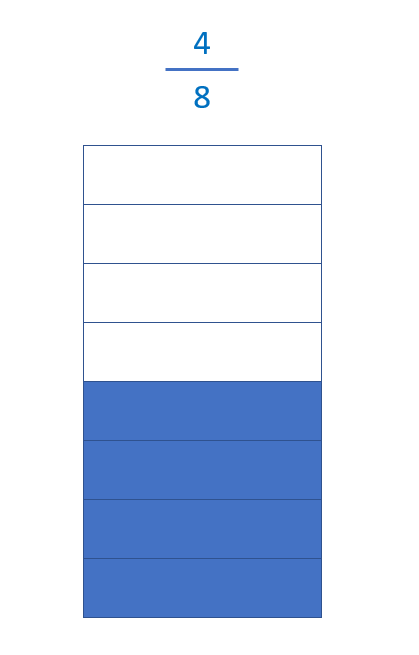When we see this fraction, we can see just by looking at it that four-eighths is the same as one half. But four-eighths is equivalent to more fractions, too. For example, the following…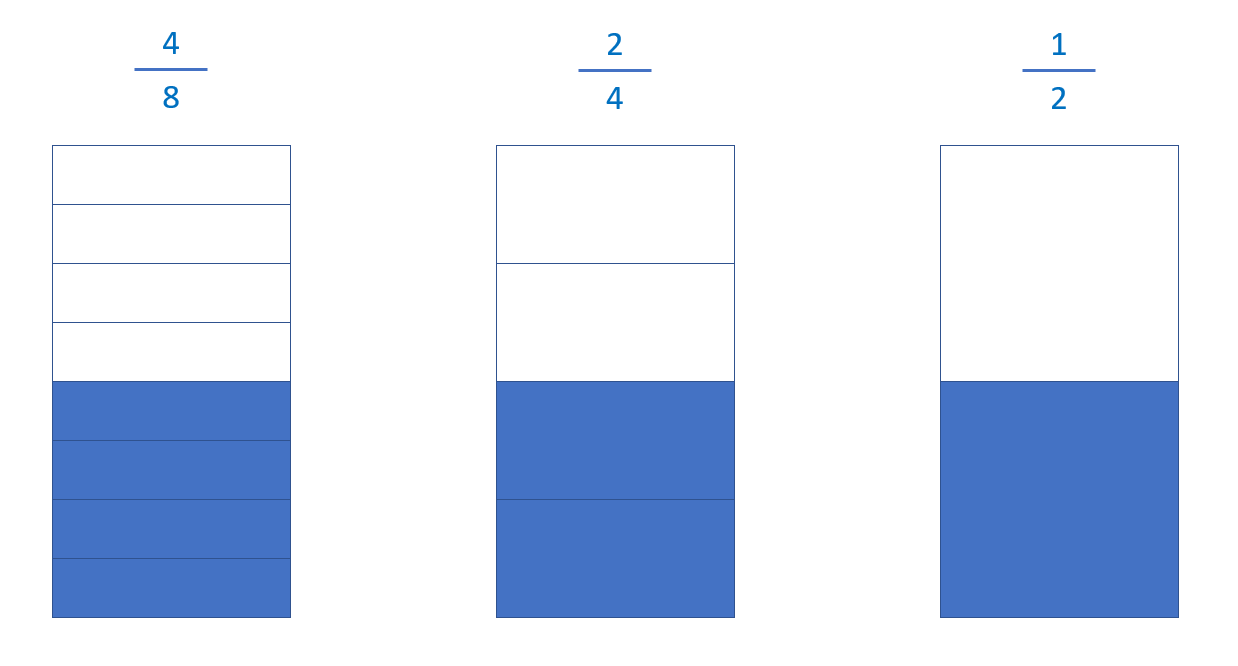#### All fractions that represent the same quantity with different values are equivalent fractions.

In this case, look closely at the two fractions on the right. They have something in common, they represent the same amount as four eighths, but with a smaller number of parts.

#### The simplified fraction of another fraction, as in the previous example, represents the same amount as the original fraction but with fewer parts.

Now we’re going to learn how to simplify a fraction mathematically.

We have to divide the numerator (4) and the denominator (8) of the original fraction by the same number. In this case, we divide them both by 2. The fraction that we get as a result is a simplified version of the previous one.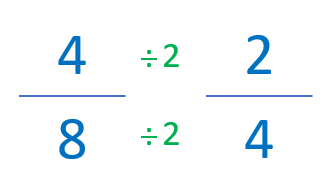We can keep simplifying our original fraction to try and find an even simpler one. So, if we divide by a bigger number (4), the result is one half.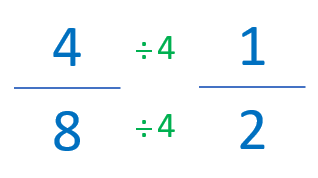If we put these fractions in a sequence, we can see that a half is also a simplified fraction of two quarters.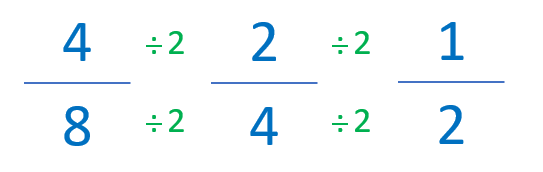Finally, what happens when we find ourselves with a fraction that we can’t simplify? For example, a half?

#### Fractions that can’t be simplified are called irreducible fractions.

I hope you’ve enjoyed learning how to simplify fractions!